### Day 16 - Rates of Change - 01.29.15

Update

Bell Ringer

1. Find the derivative of the following: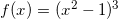1.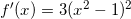2.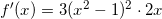3.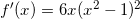4.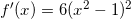5. none of the above

Review
• Prerequisite
• Secant Lines (video)
• Tangent Lines (video)
• Equation of a Tangent Line (video)
• Derivative (video)
• Derivative Rules
• Constant Rule (video)
• Power Rule (video)
• Constant Multiple Rule (video)
• Sum and Difference Rule (video)
• Derivative of Sine and Cosine Functions
• Higher Order Derivatives
• Rates of Change
• Position Function
• Velocity Function
• Acceleration Function
• Product Rule (video)
• Quotient Rule (video)

Lesson
• Work Day

Exit Ticket
• Posted on the board at the end of the block
Lesson Objectives
• How are the derivatives of products and quotients calculated?

#### In-Class Help Requests

Standard(s)
• APC.5
• Investigate derivatives presented in graphic, numerical, and analytic contexts and the relationship between continuity and differentiability.
• The derivative will be defined as the limit of the difference quotient and interpreted as an instantaneous rate of change.
• APC.6
• ​The student will investigate the derivative at a point on a curve.
• Includes:
• finding the slope of a curve at a point, including points at which the tangent is vertical and points at which there are no tangents
• using local linear approximation to find the slope of a tangent line to a curve at the point
• ​defining instantaneous rate of change as the limit of average rate of change
• approximating rate of change from graphs and tables of values.
• APC.7
• Analyze the derivative of a function as a function in itself.
• Includes:
• comparing corresponding characteristics of the graphs of f, f', and f''
• ​defining the relationship between the increasing and decreasing behavior of f and the sign of f'
• ​translating verbal descriptions into equations involving derivatives and vice versa
• defining the relationship between the concavity of f and the sign of f "
• APC.9
• Apply formulas to find derivatives.
• Includes:
• derivatives of algebraic and trigonometric functions
• derivations of sums, products, quotients, inverses, and composites (chain rule) of elementary functions
• derivatives of implicitly defined functions
• higher order derivatives of algebraic and trigonometric functions

Past Checkpoints